# FINAL

25 de Feb de 2010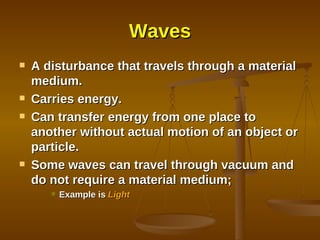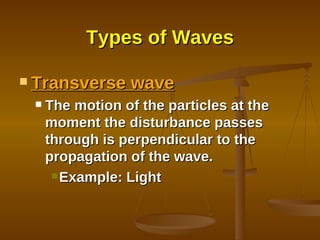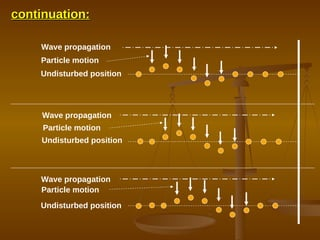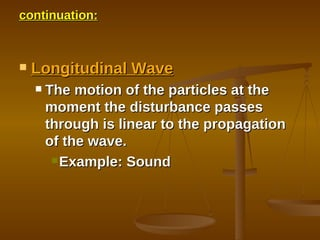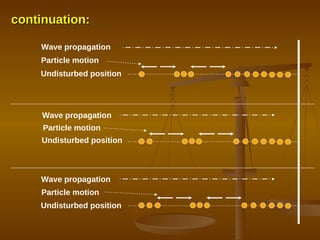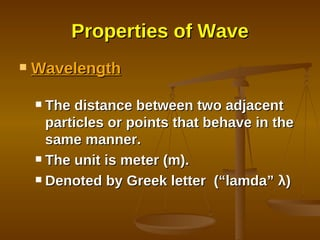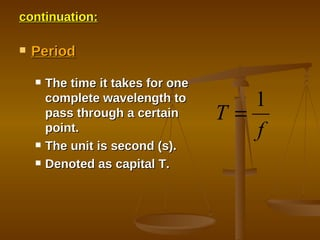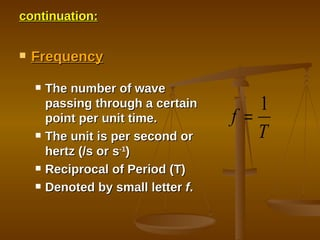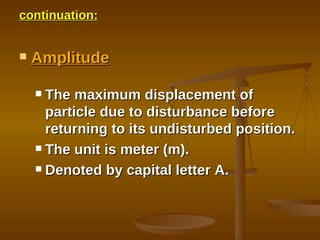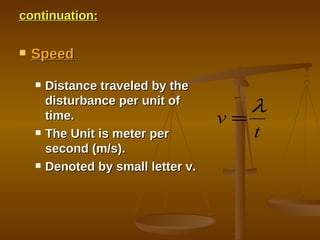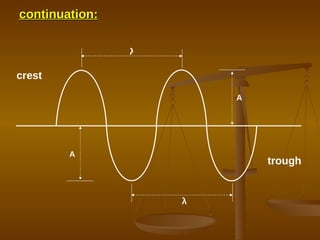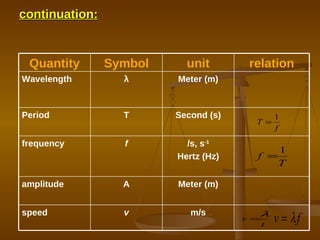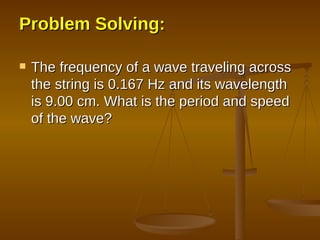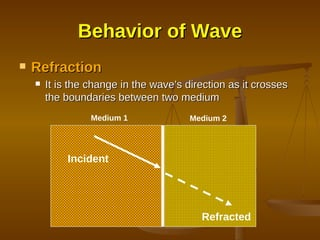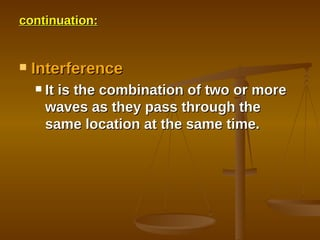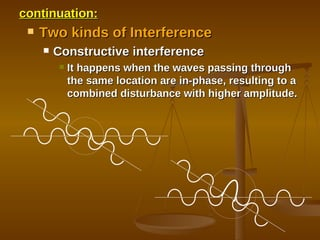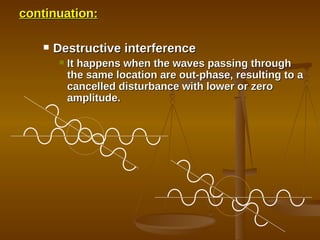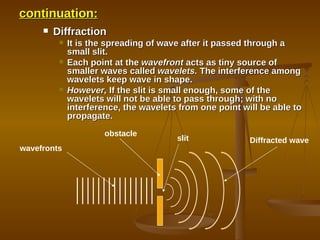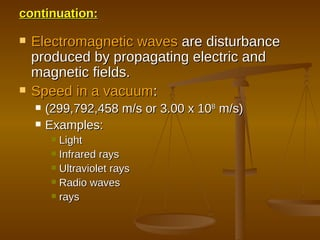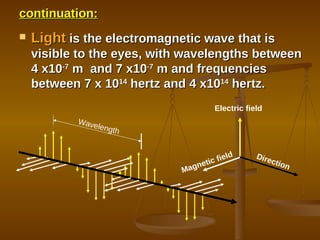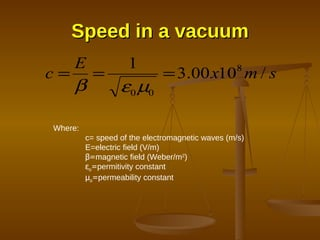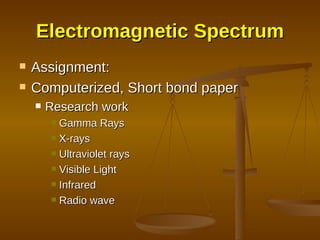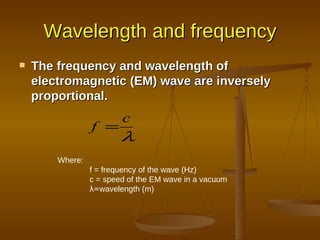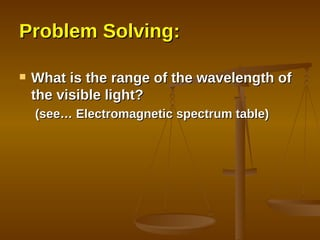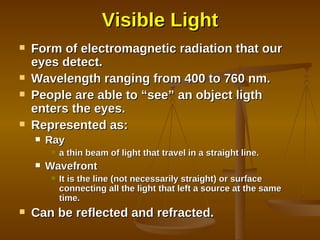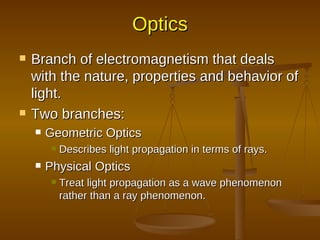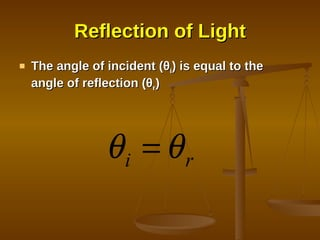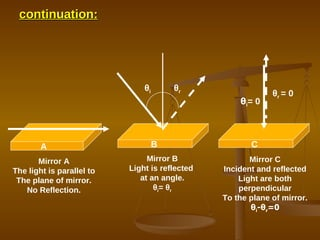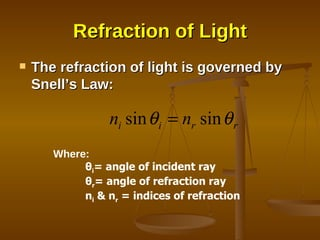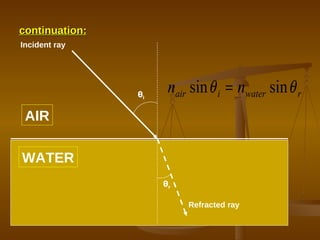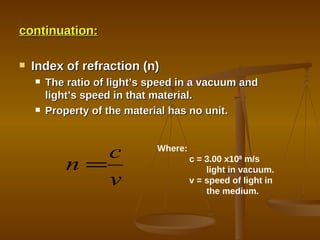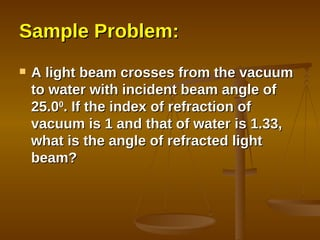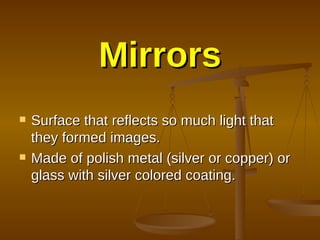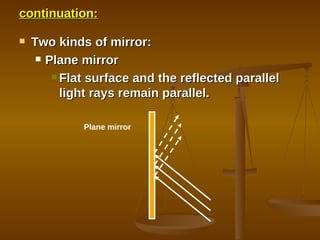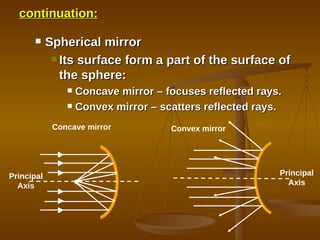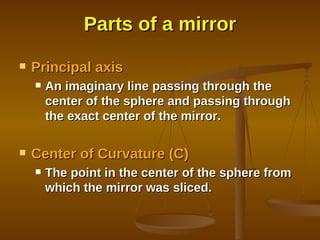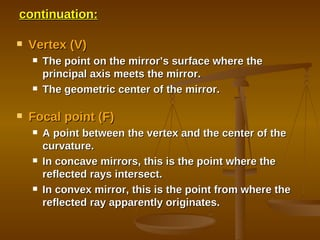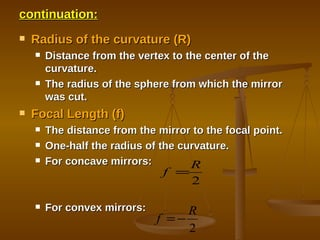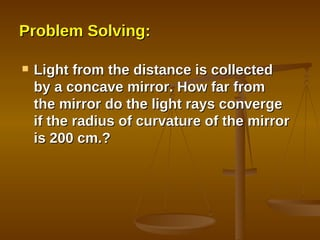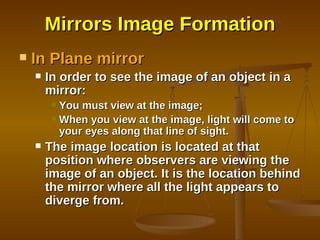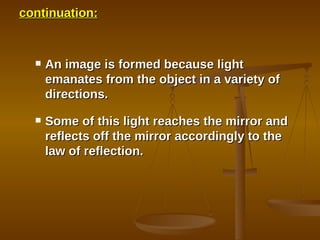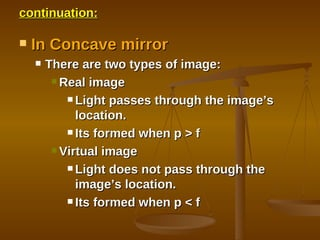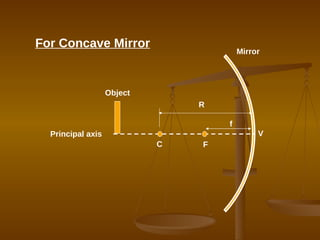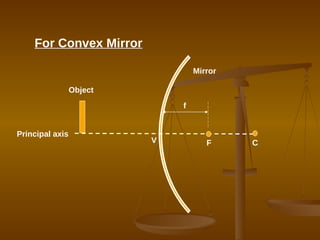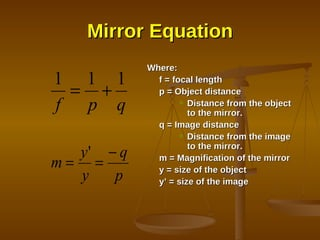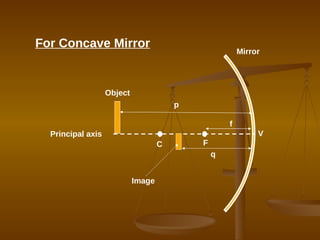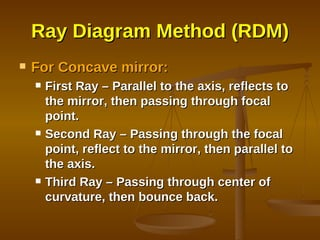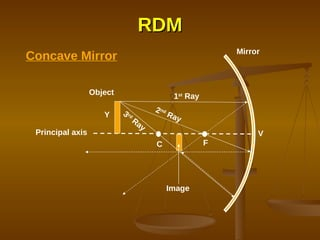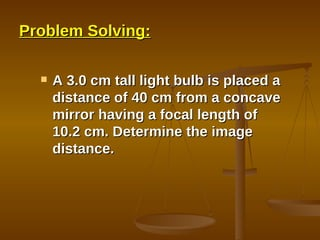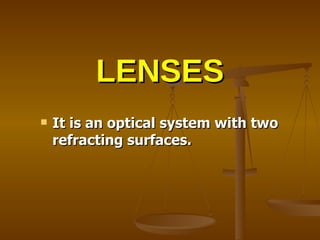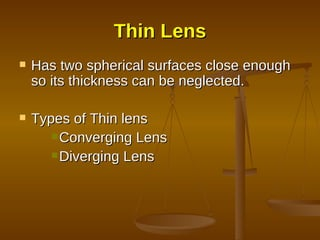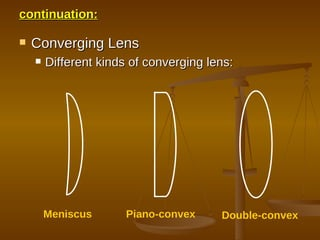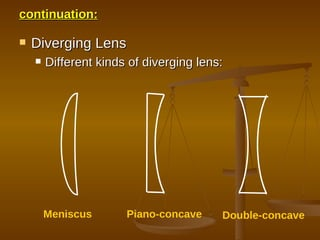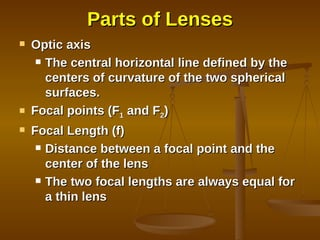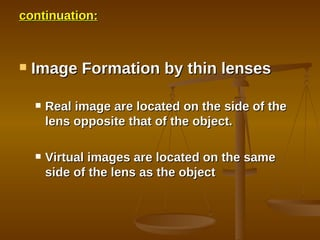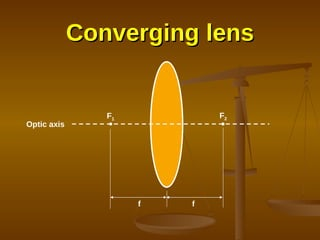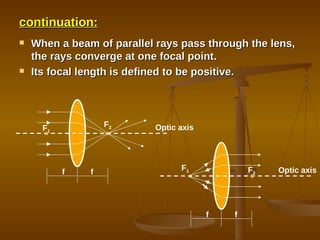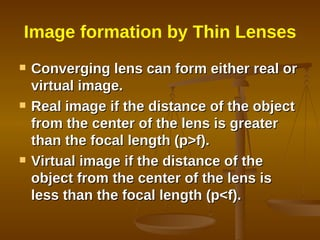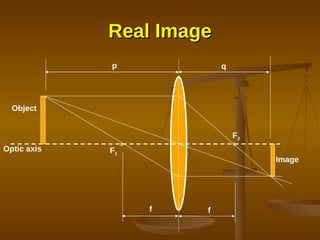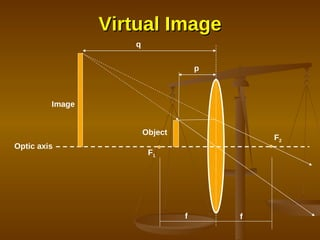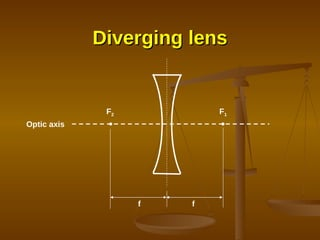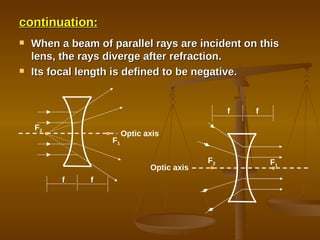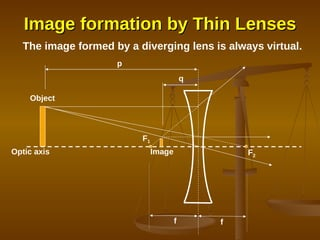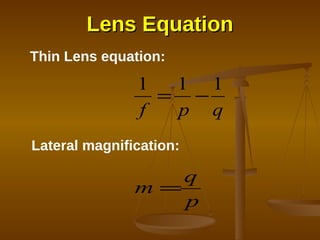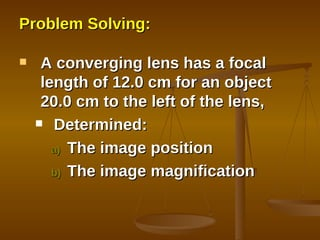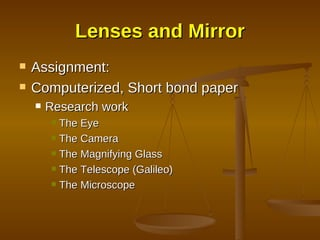1 de 72

### FINAL

• 1. Nature of Waves
• 2.
• 3.
• 4. continuation: Wave propagation Wave propagation Wave propagation Particle motion Particle motion Particle motion Undisturbed position Undisturbed position Undisturbed position
• 5.
• 6. continuation: Wave propagation Wave propagation Wave propagation Particle motion Particle motion Particle motion Undisturbed position Undisturbed position Undisturbed position
• 7.
• 8.
• 9.
• 10.
• 11.
• 12. continuation: λ λ A A crest trough
• 13. continuation: m/s v speed Meter (m) A amplitude /s, s -1 Hertz (Hz) f frequency Second (s) T Period Meter (m) λ Wavelength relation unit Symbol Quantity
• 14.
• 15.
• 16.
• 17.
• 18.
• 19.
• 20.
• 21.
• 22.
• 23.
• 24. Speed in a vacuum Where: c= speed of the electromagnetic waves (m/s) E=electric field (V/m) β = magnetic field (Weber/m 2 ) ε o = permitivity constant μ o = permeability constant
• 25.
• 26.
• 27.
• 28.
• 29. Visible Light
• 30.
• 31.
• 32.
• 33. continuation: θ i θ r θ i = 0 θ r = 0 Mirror A The light is parallel to The plane of mirror. No Reflection. Mirror B Light is reflected at an angle. θ i = θ r A B Mirror C Incident and reflected Light are both perpendicular To the plane of mirror. θ i - θ r =0 C
• 34.
• 35. continuation: AIR WATER Incident ray Refracted ray θ i θ r
• 36.
• 37.
• 38.
• 39.
• 40.
• 41.
• 42.
• 43.
• 44.
• 45.
• 46.
• 47.
• 48.
• 49. V Mirror C F For Concave Mirror Principal axis R f Object
• 50. V Mirror C F For Convex Mirror Principal axis f Object
• 51.
• 52. V Mirror C For Concave Mirror Principal axis p f Object q F Image
• 53.
• 54. RDM V Mirror C Principal axis Y F Image 1 st Ray 2 nd Ray 3 rd Ray Object Concave Mirror
• 55.
• 56.
• 57.
• 58.
• 59.
• 60.
• 61.
• 62. Converging lens F 1 F 2 f f Optic axis
• 63.
• 64.
• 65. Real Image F 1 F 2 Object Image f f p q Optic axis
• 66. Virtual Image F 1 Object F 2 f f Image p q Optic axis
• 67. Diverging lens F 1 F 2 f f Optic axis
• 68.
• 69. Image formation by Thin Lenses F 1 F 2 Object Image f f p q The image formed by a diverging lens is always virtual. Optic axis
• 70. Lens Equation Thin Lens equation: Lateral magnification:
• 71.
• 72.